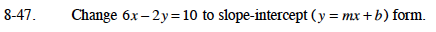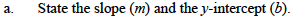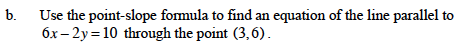### Home > CAAC > Chapter 8 > Lesson 8.2.1 > Problem8-47

8-47.6x − 2y = 10
−2y = −6x + 10
y = 3x − 5

Read the slope and the y-intercept from the equation.

slope = 3
y-intercept = (0, −5)Parallel lines have the same slope.

Substitute the slope you found and the point given, (h, k) into the point-slope formula: yk = m(xh)

Simplify the equation and write in slope intercept form.

y = 3x − 3